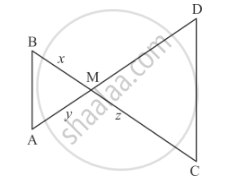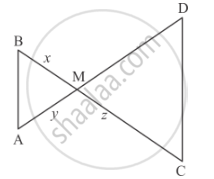Advertisement Remove all ads

# In the Given Figure, ∆Amb ∼ ∆Cmd; Determine Md in Terms of X, Y and Z. - Mathematics

Sum

In the given figure, ∆AMB ∼ ∆CMD; determine MD in terms of xy and z.Advertisement Remove all ads

#### Solution

We are given ∆AMB ∼ ∆CMD

We have to determine the value of MD in terms of x, y and z.Given  Δ AMB ∼ Δ CMD

$\Rightarrow \frac{BM}{MD} = \frac{AM}{CM}$
$\frac{x}{MD} = \frac{y}{z}$

By cross multiplication we get  MD = (xz)/y

Hence, the value of MD is  (xz)/y.

Concept: Triangles Examples and Solutions
Is there an error in this question or solution?
Advertisement Remove all ads

#### APPEARS IN

RD Sharma Class 10 Maths
Chapter 7 Triangles
Q 10 | Page 125
Advertisement Remove all ads

#### Video TutorialsVIEW ALL 

Advertisement Remove all ads
Share
Notifications

View all notifications

Forgot password?
Course